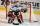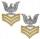# Circles

How many different circles is determined by 9 points at the plane, if 6 of them lie in a straight line?

Result

n =  64

#### Solution:Leave us a comment of example and its solution (i.e. if it is still somewhat unclear...):Be the first to comment!#### To solve this example are needed these knowledge from mathematics:

Would you like to compute count of combinations?

## Next similar examples:

1. Points in planeThe plane is given 12 points, 5 of which is located on a straight line. How many different lines could by draw from this points?
2. LinesIn how many points will intersect 14 different lines, where no two are parallel?
3. TrinityHow many different triads can be selected from the group 43 students?
4. Fish tankA fish tank at a pet store has 8 zebra fish. In how many different ways can George choose 2 zebra fish to buy?
5. HockeyHockey match ended 8:2. How many different matches could be?
6. ChordsHow many 4-tones chords (chord = at the same time sounding different tones) is possible to play within 7 tones?On the menu are 12 kinds of meal. How many ways can we choose four different meals into the daily menu?
8. First classThe shipment contains 40 items. 36 are first grade, 4 are defective. How many ways can select 5 items, so that it is no more than one defective?
9. CombinationsFrom how many elements we can create 990 combinations 2nd class without repeating?
10. ExaminationThe class is 21 students. How many ways can choose two to examination?
11. BlocksThere are 9 interactive basic building blocks of an organization. How many two-blocks combinations are there?
12. Theorem proveWe want to prove the sentence: If the natural number n is divisible by six, then n is divisible by three. From what assumption we started?
13. Calculation of CNCalculate: ?
14. Parametric equationFind the parametric equation of a line with y-intercept (0,-4) and a slope of -2.
15. CardsSuppose that are three cards in the hats. One is red on both sides, one of which is black on both sides, and a third one side red and the second black. We are pulled out of a hat randomly one card and we see that one side of it is red. What is the probabi
16. LineIt is true that the lines that do not intersect are parallel?
17. CardsThe player gets 8 cards of 32. What is the probability that it gets a) all 4 aces b) at least 1 ace